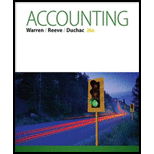Chapter 1, Problem 1.12EX### Accounting (Text Only)

26th Edition
Carl Warren + 2 others
ISBN: 9781285743615

#### Solutions

Chapter
Section### Accounting (Text Only)

26th Edition
Carl Warren + 2 others
ISBN: 9781285743615
Textbook Problem
28 views

# TransactionsThe following selected transactions were completed by Cota Delivery Service during July: 1. Received cash from owner as additional investment, $35,000. 2. Purchased supplies for cash,$1,100. 3. Paid rent for October, $4,500. 4. Paid advertising expense,$900. 5. Received cash for providing delivery services, $33,000. 6. Billed customers for delivery services on account,$58,000. 7. Paid creditors on account, $2,900. 8. Received cash from customers on account,$27,500. 9. Determined that the cost of supplies on hand was $300 and$8,600 of supplies had been used during the month. 10. Paid cash to owner for personal use, $2,500. Indicate the effect of each transaction on the accounting equation by listing the numbers identifying the transactions, (1) through (10), in a column, and inserting at the right of each number the appropriate letter from the following list: a. Increase in an asset, decrease in another asset. b. Increase in an asset, increase in a liability. c. Increase in an asset, increase in owner’s equity. d. Decrease in an asset, decrease in a liability. e. Decrease in an asset, decrease in owner’s equity. To determine Accounting equation: Accounting equation is an accounting tool expressed in the form of equation, by creating a relationship between the resources or assets of a company, and claims on the resources by the creditors and the owners. Accounting equation is expressed as shown below: Assets = Liabilities + Owners' Equity To indicate: The effect of given transactions on accounting equation Explanation 1. 1. (c) Received cash from owner as additional investment,$35,000. In assets cash increases by $35,000; Owners’ equity increases by$35,000.
1. 2. (b) Purchased supplies for cash, $1,100. In assets cash decreases by$1,100, while supplies increases by $1,100. 1. 3. (b) Paid rent for October,$4,500. In assets cash decreases by $4,500, while prepaid rent increases by$4,500.
1. 4. (e) Paid advertising expense, $900. In assets cash decreases by$900, while owner's equity decreases by $900. 1. 5. (c) Received cash for providing delivery services,$33,000. In assets cash increases by $33,000, while owner's equity increases by$33,000.
1. 6. (c) Billed customers for delivery services on account, \$58,000...

### Still sussing out bartleby?

Check out a sample textbook solution.

See a sample solution

#### The Solution to Your Study Problems

Bartleby provides explanations to thousands of textbook problems written by our experts, many with advanced degrees!

Get Started